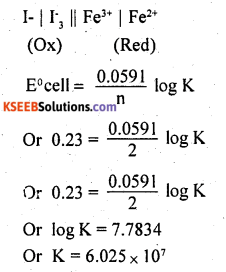# 2nd PUC Chemistry Question Bank Chapter 3 Electrochemistry

## Karnataka 2nd PUC Chemistry Question Bank Chapter 3 Electrochemistry

### 2nd PUC Chemistry Electrochemistry NCERT Textbook Questions and Answers

Question 1.
Arrange the following metals in the order in which they displace each other from the solution of their salts.
Al, Cu, Fe, Mg and Zn.
Mg, Al, Zn, Fe, Cu.

Question 2.
Given the standard electrode potentials, K+/K = -2.93V, Ag+/Ag = 0.80V,
Hg2+/Hg = 0.79 V
Mg2+/Mg = -2,37 V, Cr3+/Cr = – 0.74V
Arrange these metals in their increasing order of reducing power.
It may be noted that the lesser the E° value for an electrode, the more will be reducing power. The increasing order of reducing power is Ag+/Ag < Hg2+/Hg < Cr3+/Cr < Mg2+/Mg < K+/K

Question 3.
Depict the galvanic cell in which the reaction Zn(s)+2Ag+(aq) → Zn2+(aq)+2Ag(s) takes place. Further show:
(i) Which of the electrode is negatively charged?
(ii) The carriers of the current in the cell.
(iii) Individual reaction at each electrode.
The galvanic cell for the reaction is
Zn(s) | Zn2+(aq) || 2Ag+(aq) | 2Ag(s)
(i) Zinc electrode (anode) is negatively charged.
(ii) From silver to zinc in external circuit
(iii) Anode: Zn (s) → Zn2+(aq) + 2e
Cathode: 2Ag+(aq) + 2e → 2Ag(s)Question 4.
Calculate the standard cell potentials of galvanic cell in which the following reactions take place:
(i) 2Cr(s) + 3Cd2+(aq) → 2Cr3+(aq) + 3Cd
(ii) Fe2+(aq) + Ag+(aq) → Fe3+(aq) + Ag(s)
Calculate the ArG9and equilibrium constant of the reactions.
(i) For the given reaction, the Nemst equation can be given as: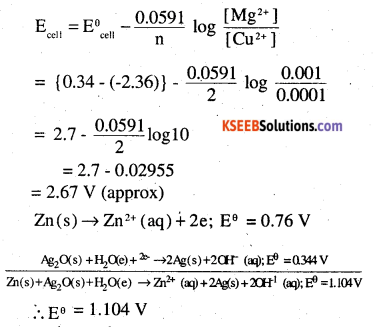∴ Eθ = 1.104V
We know that,
ΔrGθ = -nFEθ
= -2 × 96487× 1.04
= – 213043.296J
= -213.04KJ

Question 5.
Write the Nernst equation and em! of the
following cells at 298 K:
(I) Mg(s)|Mg+2(O.OO1M) ||Cu+2(0.0001M)|Cu(s) .
(ii) Fe(s)|Fe+2(O.OO1 M)||H+(1M)H2(g) (1bar)|Pt(s)
(iii) Sn(s) |Sn2+(O.050 M)||H+(0.020M|H2(g) (1 bar)|Pt(s)
(iv) Pt(s)|Br2(l)|Br(O.O1O M)||H+(O.030 M)| H2(g) (1 bar)|Pt(s).
(i) EθCr3+ /Cr = 0.74V
EθCd2+ / Cd = – 0.40V
The galvanic cell of the reaction IC depicted as :
Cr(s)|Cr3+ (aq) || (Cd2+ (aq) Cd(s)
Now, the standard cell potential is
Eθcell = EθR– EθL
= -40 – (-0.74)
= +0.34V
rGθ = —nFEθcell
In the given equation,
n = 6
F = 96487 C mol-1
Eθcell = + 0.34V
Then, ∆rGθ =-6 × 96487 mol-1 × 0.34V
= -196833.48 CV mol-1
= -196833.48 J mol-1
= -196.83 KJ mol-1
Again ∆rGθ = -RT In K
⇒ ∆rGθ =-2.303RT log K(ii) Eθ Fe3+ /Fe2+ = 0.77V
Eθ Ag/Ag = 0.80V
(ii) For the given reaction, the Nernst equation can be given as: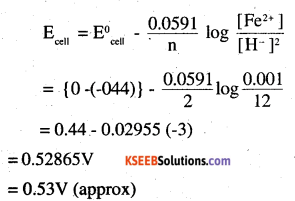(iii) Far the givën reaction, the Nernst equation can be given as: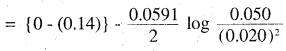0.14 – 0.0295 × log 125
= 0.14-0.062
= 0.078 V
= 0.08 V (approx)

(iv) For the given reaction , the nernst equation can be given as: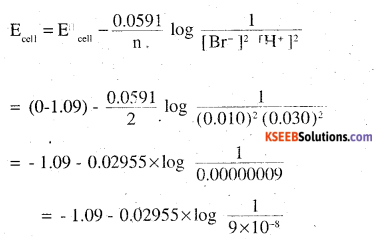= -1.09 – 0.02955 × log ( 1.11× 107)
= -1.09 – 0.02955(0.0453 + 7)
= -1.09 – 0.208
= -1.298 V.Question 6.
In the button cells widely used in watches and other devices the following reaction takes place:
Zn(s) + Ag2O(s) + H2O(l) → Zn2 + (aq) + 2Ag(s) + 2OH-(aq)
Determine ∆r Gθ and Eθ for the reaction.
The galvanic cell of the reaction is depicted as:
Fe2+ (aq) | Fe3+ (aq) || Ag+ (aq) | Ag(s)
Now, the standard cell potential is
Eθcell = EθR – Elθ
= 0.80 – 0.77 ‘
= 0.03 V
Here, n = 1
Then, ∆r Gθ = – nFEθcell
= -1 × 96487 C mol-1 × 0.03V
= -2894.61 J mol-1
= -2.89 KJ mol-1
r Gθ = 2.303 RT ln KQuestion 7.
Define conductivity and molar conductivity for the solution of an electrolyte.
Discuss their variation with concentration.
The conductivity of a solution is defined as the conductance of a solution of 1 cm length and having 1 sq. cm as the area of cross-section. Molar conductivity of a solution at a dilution (V) is the conductance of all the ions produced from one mole of the electrolyte dissolved in V cm3 of the solution when the electrodes are one cm apart and the area of cross-section of the electrodes is so large that the whole of the solution is contained between them. It is usually represented by Λm.

The conductivity of a solution (both for strong and weak electrolytes) decreases with a decrease in the concentration of the electrolyte, i.e., on dilution. This is due to the decrease in the number of ions per unit volume of the solution on ‘ dilution. The molar conductivity of a solution increases with a decrease in the concentration of the electrolyte. This is because both the number of ions, as well as mobility of ions, increases with dilution. When concentration approaches zero, the molar conductivity is known as limiting molar conductivity.

Question 8.
The conductivity of 0.20 M solution of KCl at 298 K is 0.0248 S cm-1. Calculate its molar conductivity.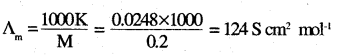Question 9.
The resistance of a conductivity cell containing 0.001M KCl solution at 298 K is 1500Ω. What is the cell constant if the conductivity of 0.001M KCl solution at 298 K is 0.146 × 10-3 S cm-1.
Cell constant = conductivity × Resistance
= 0.146 × 10-3S Cm-1 × 1500 Ω
= 0.219 cm-1Question 10.
The conductivity of sodium chloride at 298 K has been determined at different concentrations and the results are given below:Calculate ∆m for all concentrations and draw a plot between ∆m and c1/2. Find the value of ∆0m
K = 7.896 × 10-5 S cm-1
M = 0.00241Question 11.
How much charge is required for the following reductions?
(i) 1 mol of Al3+ to Al
(ii) 1 mol of Cu2+ to Cu
(iii) 1 mol of MnO4- to Mn2+
Al3+ + 3e → A1
charge required = 3F
(ii) Cu2+ + 2e → Cu
charge required = 2F
(iii) MnO4- + 8H+ + Se → Mn2+ + H2O
charge required = 5F

Question 12.
How much electricity in terms of Faraday ¡s required to produce
(j) 20.0 g at Ca from molten CaCl2
(ii) 40.0 g of Al from Almólten Al2O3
(i) Ca2+2 + 2e → Ca
2F can produce I mole (=40 g) Ca
∴ To produce 20 g Ca requires, $$\frac{2 \mathrm{F} \times 20}{40}$$ = 1F

(ii) Al3+ + 3e → Al
3F can produce 1 mole (= 27g) Al
∴ To produce 40 g A1 requires
$$\frac{3 \mathrm{F} \times 40}{27}$$ = 4.44F

Question 13.
How much electricity is required in coulomb for the oxidation of
(i) 1 mol of H2O to O2
(ii) 1 mol of FeO to Fe2O3.
(i) 2H2O → 4H+ + O2 + 4e
2F of electricity is required for oxidation of 1 mole of H2O
(ii) Fe2+ → Fe3+ + e
IF of electricity is required for oxidation of 1 mole FeO

Question 14.
A solution of Ni(NO3)2 is electrolysed between platinum electrodes using a current of 5 amperes for 20 minutes. What mass of Ni is deposited at the cathode?
Ni2+ + 2e → Ni
2F (2 × 96500 C) can produce 58.7 g of Ni
Q = It = 5 × 20 × 60 =6000C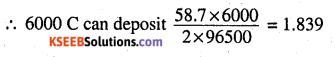Question 15.
Three electrolytic cells A,B,C containing = 0.439 g of Zn solutions of ZnSO4, AgNO3 and CuSO4, respectively are connected in series. A steady current of 1.5 amperes was passed through them until 1.45 g of silver deposited at the cathode of cell B. How long did the current flow? What mass of copper and zinc were deposited?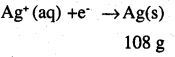i.e. 108 g of Ag is deposited by 96487 C
Therefore, 1 .45g of Ag is deposited byGiven,
Current = 1.5A
$$\frac{1295.43}{1.5}$$ S
∴ Time = 863.6S
= 864 S
= 14.40 min
Again,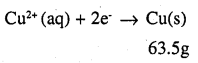i.e. 2 × 96487 C of charge deposit = 63.5 g of Cu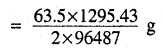Therefore, 1295.43 C of charge will deposit = 0.426g of Cui.e. 2 × 96487 C of charge deposit = 65.4 g of Zn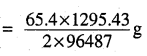Therefore, 1295.43 C of charge will deposit = 0.439 g of ZnQuestion 16.
Predict the products of electrolysis in each of the following:
(i) An aqueous solution of AgNO3 with silver electrodes.
(ii) An aqueous solution of AgNO3 with platinum electrodes.
(iii) A dilute solution of H2SO4 with platinum electrodes.
(iv) An aqueous solution of CuCl2 with platinum electrodes.
(a) At cathode : Possible reactions are :
(i) Reduction of Ag+Ag = 0.80V
(ii) Reduction of H2O E°H2O = -0.83 V
∴ Ag+ ion reduced because its E°Ag > E°H2O
At anode : Possible reactions are :
(i) Oxidation of H2O E° = 1.23 V
(ii) Oxidation of Ag E° = 0.80V
Since E°Ag < E°H2O ∴ Ag gets oxidised.

(b) Possible reactions at the cathode:
(i) Reduction of Ag+g = 0.80V
(ii) Reduction of water E°H2O = -0.8 V .
Since E°Ag > E°H2O ∴Ag+ gets reduced.
Possible reactions at anode :
Oxidation of water E°Ag = 1.23
∴ O2 gas is liberated at anode.

(c) Possible reaction at cathode:
(i) Reduction of H+H+/H2 0.0
(ii) Reduction of H2O E°H2O = -0.87
Since E°H+/H2 > E°H2O ∴ H2 gas is liberated at cathode.
Possible reaction at anode :
Oxidation of water E°H2O = 1.23
∴ O2 gas is liberated at anode.

(d) Possible reaction at cathode :
(i) Reduction of water E° H2O = -0.87V
(ii) Reduction of Cu2+ E°Cu2+/Cu = 0.34V
Since E°cu2+/cu > E°H2O ∴ Cu2+ gets reduced at cathode.
Possible reaction at anode :
(i) Oxidation of water E°H2O = 1.23V
(ii) Oxidation of Cl- E° Cl/Cl2 = 1.36V
Cl2 gas is liberated at anode because oxidation of water is kinetically slow.

Question 1.
A solution of sodium chloride is a better
conductor of electricity at a temperature of 50°C than at room temperature. Why?
A solution of NaCl shows greater conduction of electricity at a temperature of 50°C than at room temperature because the ionic mobility of a strong electrolyte such as NaCl increases with an increase in temperature.

Question 2.
Give the relationship between molar conductivity and specific conductivity.
Molar conductivity and specific conductivity are related to each other by the given equation.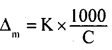Where,
Δm = Molar conductivity
K = Specific conductivity
C = Molar concentration

Question 3.
Why is it not possible to measure single electrode potential?
The process of oxidation or reduction cannot take place alone. However, electrode potential is a relative tendency and can be measured with respect to a reference electrode such as standard hydrogen electrode.

Question 4.
Why is the rusting of iron faster in saline water than in pure water?
Strong electrolytes such as sodium chloride are present in saline water. The ions produced from NaCl help in the reduction of oxygen to form water. Hence, the rusting of iron is faster in saline water than in pure water.

Question 5.
What happens Δ0m for weak electrolytes obtained by using Kohlrausch law if the migration of ions is increased to three
Δ0m for weak electrolytes obtained by using Kohlrausch law is independent of the migration of ions.Question 6.
During a classroom discussion about electrochemical cells, Raju said that an electrochemical cell cannot work without a salt bridge?

1. How far is the above statement true?
2. What do you understand by a salt bridge?
3. Explain the important uses of a salt bridge?

1. Raju’s statement is correct. A salt bridge completes the electrical circuit by connecting the electrode solution externally.
2. Salt bridge is a U-shaped glass tube filled with a jelly-like substance, agar-agar, mixed with an electrolyte like KCl. KNO3, etc.
• It permits electrical contact between the electrode solutions but prevents mixing.
•  It maintains the electrical neutrality of the solution by inter-migration of ions into two half cells.
• It reduces the so-called liquid – junction potential (Voltage produced where two dissimilar solutions are in contact).
• The salt bridge provides a sufficient amount of cations and anions to replace the ions lost or produced in the two half cells.

Question 7.

1. In lab work, Ramu noticed that solid NaCl does not conduct electricity while NaCl solution can conduct electricity. If he asked for the above, how will you explain it?
2. How many coulombs are required for the following conversions?

a. 1 mole of Al3+ to Al
b. 1 mole of Cu2+ to Cu
c. 1 mole of MnO4 to Mn2+?
1. The conduction of electricity in electrolytes arises due to the movement of ions. The ions of an electrolyte in the solid-state occupy fixed lattice points. The ions in solid electrolytes cannot move from one point to another. Due to the lack of movement of ions, it does not conduct electricity.

2. a. 1 mole of Al3+ to Al
Al3+ + 3e → Al
i.e., 1 mole of Al requires 3 moles of electrons or 3 × 96500 C
= 289500 C

b. 1 mole of Cu2+ to Cu
Cu2+ + 2e → Cu
i.e., 1 mole of Cu requires 2 moles of electrons = 2 × 96500 C
= 193000 C

c. 1 mole of MnO4 to Mn2+
MnO4 + 8H+ + 5e → Mn2+ + 4H2O
∴ Conversion of 1 mole of MnO4 to Mn2+ requires 5 moles of electrons
= 5 × 96500 C
= 482500 C

Question 8.
The electrolysis of a salt solution of a metal was carried out by passing a current of 4A for 45 minutes. This resulted in the deposition of 2.977g of the metal. If the atomic mass of the metal is 106.4 g mol-1, then calculate the charge present in the metal cation.
Let the charge on the metal cation be h i.e. the metal cation is M+
Accordingly,
Mh+ + 4e → M
Therefore, a current of h × 96500 coulomb will deposit 106.4 g of metal quantity of charge passed = 10800 coulombs
Now, 10800 coulombs will deposit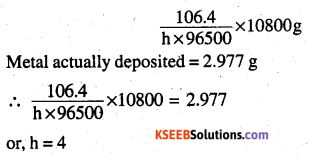Hence, the charge present on the metal cation is + 4.

Question 9.
(a) At infinite dilution, the ionic conductance of Ba2+ and Cl is 121 and 76 ohm1 cm respectively. What will be the equivalent
conductance of BaCl2 (in ohm-1 cm2) at infinite dilution?
(b) What effect does concentration have on the molar conductivity of a strong electrolyte?
(a) The molar conductivity of barium chloride is given by the following equation:
Δ0m(BaCl2) = Δ0Ba2+ + 2 Δ0Cl-
= 127 + 2 × 76 = 279Ω-1 cm2 mol-1
Δ0 eq = $$\frac{279}{2}$$ Ω-1cm2Eq-1
= 139.5 Ω-1cm2 Eq-1
[∴ Eq.wt. of BaCl2 = $$\frac{1}{2}$$ × mol.wt]
Hence the equivalent conductance of BaCl2 at infinite dilution is 139.5 Ω-1cm2 eq-1

(b) The molar conductivity of a strong electrolyte decreases with the square root of concentration in linear fashion, as shown below.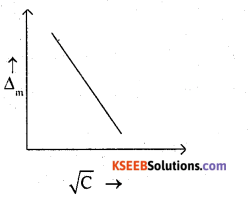Question 10.
(a) The standard reduction potentials of Fe3+ | Fe2+ and I3 | Iare 0.77 V and 0.54 V respectively for the reaction 2Fe3+ + 3I ⇌ 2Fe2+ + I3. Calculate the value of equilibrium constant.
(b) How much charge is required 1 mole of Cu2+ to Cu